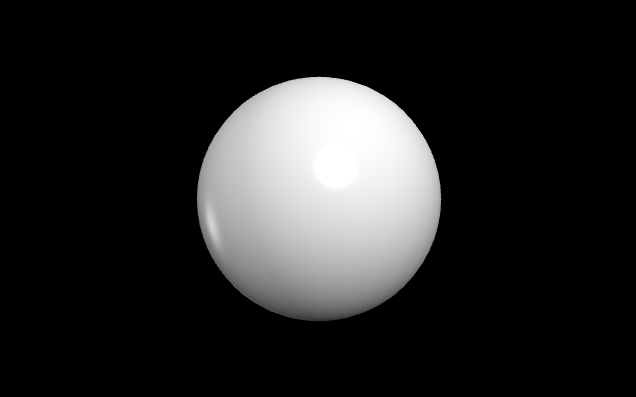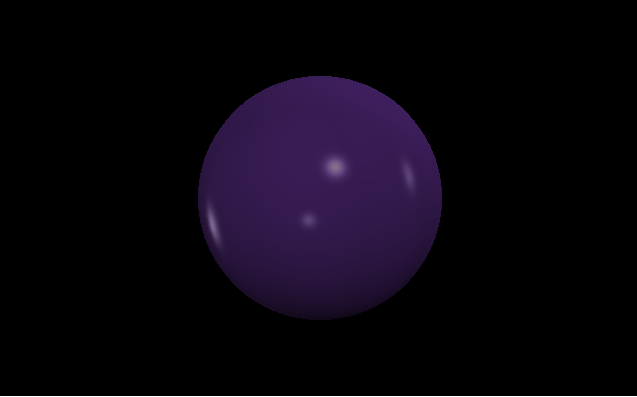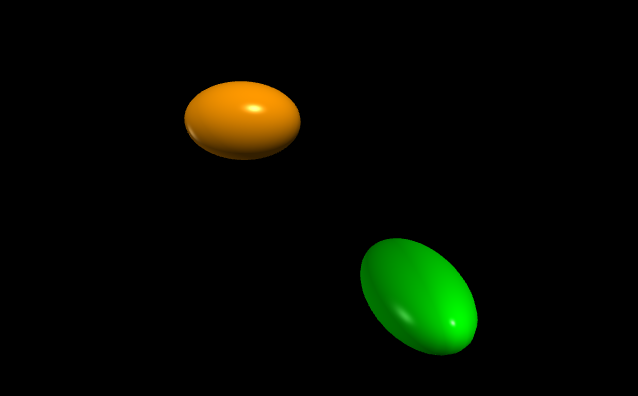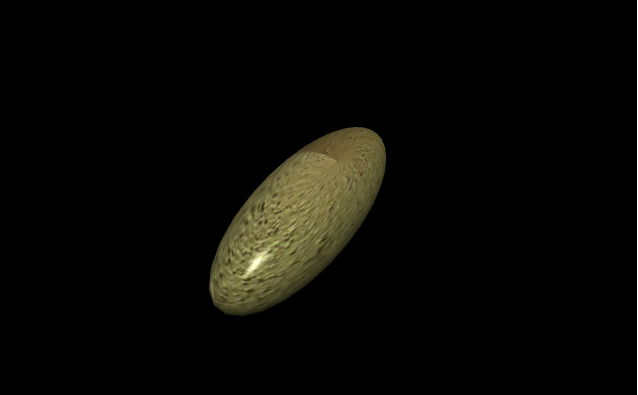Related Articles

# Making an ellipsoid with VPython

• Last Updated : 08 Jun, 2020

`VPython` makes it easy to create navigable 3D displays and animations, even for those with limited programming experience. Because it is based on Python, it also has much to offer for experienced programmers and researchers. `VPython` allows users to create objects such as spheres and cones in 3D space and displays these objects in a window. This makes it easy to create simple visualizations, allowing programmers to focus more on the computational aspect of their programs. The simplicity of `VPython` has made it a tool for the illustration of simple physics, especially in the educational environment.

Installation :

`pip install vpython`

An ellipsoid is a geometrical object in three-dimensional space in which all plane cross sections are either ellipses or circles forming a closed surface. We can generate an ellipsoid in `VPython` using the `ellipsoid()` method.

## ellipsoid()

Syntax : ellipsoid(parameters)

Parameters :

• pos : It is the position of the center of the ellipsoid. Assign a vector containing 3 values, example pos = vector(0, 0, 0)
• axis : It is the axis of alignment of the ellipsoid. Assign a vector containing 3 values, example axis = vector(1, 2, 1)
• up : It is the orientation of the ellipsoid. Assign a vector containing 3 values, example up = vector(0, 1, 0)
• color : It is the color of the ellipsoid. Assign a vector containing 3 values, example color = vector(1, 1, 1) will give the color white
• opacity : It is the opacity of the ellipsoid. Assign a floating value in which 1 is the most opaque and 0 the least opaque, example opacity = 0.5
• shininess : It is the shininess of the ellipsoid. Assign a floating value in which 1 is the most shiny and 0 the least shiny, example shininess = 0.6
• emissive : It is the emissivity of the ellipsoid. Assign a boolean value in which True is emissive and False is not emissive, example emissivity = False
• texture : It is the texture of the ellipsoid. Assign the required texture from the textures class, example texture = textures.stucco
• length : It is the length of the ellipsoid in x direction. Assign a floating value, the default length is 1, example length = 10
• height : It is the height of the ellipsoid in y direction. Assign a floating value, the default height is 1, example height = 8
• width : It is the width of the ellipsoid in z direction. Assign a floating value, the default width is 1, example width = 5
• size : It is the size of the ellipsoid. Assign a vector containing 3 values representing the length, height and width respectively, example size = vector(1, 1, 1)

All the parameters are optional.

Example 1 :An ellipsoid with no parameters, all the parameters will have the default value.

 `# import the module``from` `vpython ``import` `*` `ellipsoid()`

Output :Example 2 :An ellipsoid using the parameters color, opacity, shininess and emissivity.

 `# import the module``from` `vpython ``import` `*` `ellipsoid(color ``=` `vector(``0.4``, ``0.2``, ``0.6``), ``          ``opacity ``=` `0.5``, ``          ``shininess ``=` `1``, ``          ``emissive ``=` `False``)`

Output :Example 3 :Displaying 2 ellipsoids to visualize the attributes pos, length, height and wisth.

 `# import the module``from` `vpython ``import` `*`` ` `# the first ellipsoid``ellipsoid(pos ``=` `vector(``-``2``, ``2``, ``0``),``           ``length ``=` `3``,``           ``height ``=` `2``,``           ``width ``=` `2``,``           ``color ``=` `vector(``1``, ``0.6``, ``0``))``  ` `# the second ellipsoid``ellipsoid(pos ``=` `vector(``1``, ``-``1``, ``5``), ``          ``width ``=` `3``,``           ``color ``=` `vector(``0``, ``1``, ``0``))`

Output :Example 4 :An ellipsoid using the parameters texture, axis and up.

 `# import the module``from` `vpython ``import` `*` `ellipsoid(texture ``=` `textures.stucco,``          ``axis ``=` `vector(``-``1``, ``4``, ``0``),``          ``up ``=` `vector(``1``, ``2``, ``2``),``          ``length ``=` `3``)`

Output :Attention geek! Strengthen your foundations with the Python Programming Foundation Course and learn the basics.

To begin with, your interview preparations Enhance your Data Structures concepts with the Python DS Course. And to begin with your Machine Learning Journey, join the Machine Learning – Basic Level Course

My Personal Notes arrow_drop_up